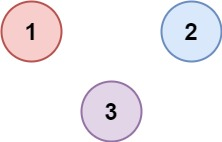# 547. Number of Provinces / Medium

There are n cities. Some of them are connected, while some are not. If city a is connected directly with city b, and city b is connected directly with city c, then city a is connected indirectly with city c.

A province is a group of directly or indirectly connected cities and no other cities outside of the group.

You are given an `n x n` matrix isConnected where `isConnected[i][j]` = 1 if the `ith` city and the `jth` city are directly connected, and `isConnected[i][j]` = 0 otherwise.

Return the total number of provinces.

## Example 1:Input: isConnected = [[1,1,0],[1,1,0],[0,0,1]]
Output: 2

## Example 2:Input: isConnected = [[1,0,0],[0,1,0],[0,0,1]]
Output: 3

## Comstraints

• 1 <= n <= 200
• n == isConnected.length
• n == isConnected[i].length
• isConnected[i][j] is 1 or 0.
• isConnected[i][i] == 1
• isConnected[i][j] == isConnected[j][i]

# Solution 1: DFS

## 效能

### Complexity

• Time Complexity: O(N^2), where N is the number of province
• Space Complexity: O(N), where N is the number of province

### LeetCode Result

• Runtime: 37 ms
• Memory Usage: 13.7 MB

# Solution 2: Union-Find (Disjoint Set)

## 效能

### Complexity

• Time Complexity: O(N^3), where N is the number of province
• Space Complexity: O(N), where N is the number of province

### LeetCode Result

• Runtime: 56 ms
• Memory Usage: 13.7 MB

# Solution 3: Union-Find (Disjoint Set) with Path Compression / Union by Rank

## 思路

Union-Find 可以透過兩個技巧來加速，透過在 Find 時將 parent[i] 指向回傳的 root，來減少遞迴時的時空間損失。

## 效能

### Complexity

• Time Complexity: O(N^2), where N is the number of province
• Space Complexity: O(N), where N is the number of province

### LeetCode Result

• Runtime: 25 ms
• Memory Usage: 13.7 MB